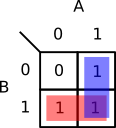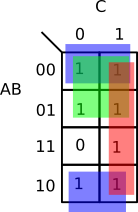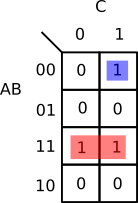# faehnri.ch

## Karnaugh Maps

### a tool for programmers

2019-12-27

Karnaugh maps are a tool for simplifying boolean expressions that can be used by programmers.

I learned about Karnaugh maps in a digital design class to simplify logic circuits. They’re a tool like state machines or logic tables, but I think they’re only taught if you’re more on the hardware side. However, I think they can be used when writing software too.

Say you have a complex condition to go into an if statement: (not A and B) or (A and B) or (A and not B).

You can perform some boolean algebra to simplify it.

``````A'B + AB + AB'
A'B + A(B + B')
A'B + A
A + A'B
``````

But there’s a chance for a mistake, and you might not have it simplified as well as it could be.

That’s where Karnaugh maps come in. They’re a way to visually represent a boolean expression in a way that you can quickly see the grouping of the statements.

The map is first constructed on a grid with columns for possible inputs of some variables, and rows for the other variables.

With this simple two-input expression above, it would first be set up like this:Each of those squares is a possible input of all the variables. The top-left is 00, or A’B’. If that’s the input into our expression, the result is 0, so we put a 0 in the top-left.

Similarly, the top right is 10 or AB’ which gets a 1. Bottom-left is 01 or A’B which is 1, and bottom-right is 11 or AB which is also 1. Our map is then:To simplify, draw rectangles around the largest groups 1s that have one, two, four, etc, 1s (powers of 2). Even if the rectangles overlap, draw the largest you can.The simplification is then the variables for each rectangle that are the same for that rectangle. For instance, the red rectangle in the above diagram covers two inputs where B is 1, the blue has inputs where A is 1.

This shows the simplification for the above boolean expression is A+B.

Our boolean algebra simplification wasn’t as simple as that. To see how we can go from A+A’B to A+B with boolean algebra:

``````A + A'B
A(1 + B) + A'B
A + AB + A'B
A + (A + A')B
A + B
``````

To further simplify, we first had to expand, and had to know what to expand with. It’s kind of like a local minima, looking around this might seem like the lowest point but too see if there’s an even lower point over the ridge you first have to climb up it. That’s where Karnaugh maps are handy, they let you see these simplifications easily.

K-maps can be used with even more variables. Consider the following truth table.You then create a map with two variables on one side. The trick though is to make it so adjacent squares only change one variable at a time. This is done with Gray codes.

Instead of counting up like in the truth table, you go through all the possible inputs but only changing by one each time.

``````00
01
10 <- this changed two
11
``````

You would have:

``````00
01
11
10
``````

Also note that it wraps from bottom to top, 10 is also one off from 00.

Our two-variable K-map was trivially in Gray code. For three variables, you could have this K-map from the above truth table:We now draw rectangles around as large groups of powers of two as possible.Note the blue rectangle is one rectangle, it just wraps around. Think of the map as a torus, it wraps top to bottom, left to right. Just like a Pac-Man level.

To simplify, we see which inputs don’t change in each rectangle. The blue rectangle has B’, green is A’, and red is C.

So the simplified expression is

``````A' + B' + C
``````

Here’s another example:This one simplifies to

``````AB + A'B'C
``````

This was a small post on Karnaugh maps just to let you know about them and so you can do a Web search for further information. These can simplify expressions in your code, but that may obscure their meaning. I suggest comments with the code to give the original intent and how you arrived at the simplified expression. Even though the code may not have the original meaning, I feel it’s still worth simplifying the expressions because that can reduce the chance of mistakes compared to writing larger expressions.